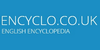Unit of angular measurement equal to the angle at the center of a circle subtended by an arc equal in length to the radius. Equals about 57.296 degrees.[n] - the unit of plane angle adopted under the System International d`UnitesSI Supplementary UnitThe radian is the plane angle between two radii of a circle which cut off on the circumference an arc equal in length to the radius.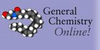(rad) Compare with steradian. An angle with vertex at the center of a circle of radius r that encompasses an arc of length r.
Found on http://antoine.frostburg.edu/chem/senese/101/glossary/r.shtmlRa'di·an (- a n) noun [ From Radius .] (Math.) An arc of a circle which is equal to the radius, or the angle measured by such an arc.
Found on http://www.encyclo.co.uk/webster/R/3noun the unit of plane angle adopted under the Systeme International d`Unites; equal to the angle at the center of a circle subtended by an arc equal in length to the radius (approximately 57.295 degrees)
Found on https://www.encyclo.co.uk/local/20974• (n.) An arc of a circle which is equal to the radius, or the angle measured by such an arc.(from the article `steradian`) ...complete surface area of a sphere is 4 times the square of its radius, the total solid angle about a point is equal to 4 steradians. Derived from ... Numerical values can be assigned to angles by selecting a unit of measure. The most common units are the degree and the radian. There are 360° in a .....
Found on http://www.britannica.com/eb/a-z/r/3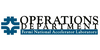A measure of angle. 360 degrees = 2p radians. The radian measure of an angle is given geometrically by the ratio arc length/radius of the arc of a circle with the angle defined at its center and subtending the arc.
Found on http://www-bdnew.fnal.gov/operations/accgloss/gloss.html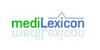(rad) Type: Term Pronunciation: rā′dē-ăn Definitions: 1. A supplementary SI unit of plane angle.
Found on http://www.medilexicon.com/medicaldictionary.php?t=74796One of two supplementary (dimensionless) units in the SI system of units (the other being the steradian). The radian is a unit of plane angle. There are 2p radians in a complete circle. One radian = 180/p degrees, or approximately 57.296°.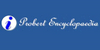The radian is the SI unit (symbol rad) of plane angles, an alternative unit to the degree. It is the angle at the centre of a circle when the centre is joined to the two ends of an arc equal in length to the radius of the circle. There are 2 pi (approximately 6.284) radians in a full circle (360 degrees). One radian is approximately 57 degrees, and...
Found on http://www.probertencyclopaedia.com/browse/AR.HTM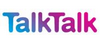SI unit of plane angles, an alternative unit to the degree. It is the angle at the centre of a circle when the centre is joined to the two ends of an arc (part of the circumference) equal in length to the radius of the circle. There are 2π (approximately 6.284) radians in a full circle (360°). One radian is approximately 57°, and 1&#...
Found on http://www.talktalk.co.uk/reference/encyclopaedia/hutchinson/m0006725.htmlThe radian is the standard unit of angular measure, used in many areas of mathematics. An angle`s measurement in radians is numerically equal to the length of a corresponding arc of a unit circle, so one radian is just under 57.3 degrees (when the arc length is equal to the radius). The unit was formerly an SI supplementary unit, but this categor....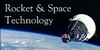Unit of angular measurement equal to the angle at the center of a circle subtended by an arc equal in length to the radius. Equals about 57.296 degrees.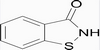An angle with vertex at the center of a circle of radius r that encompasses an arc of length r.